# Intermediate Geometry : How to find the length of the diagonal of a parallelogram

## Example Questions

### Example Question #1 : How To Find The Length Of The Diagonal Of A Parallelogram

The length of a parallelogram iscm and the width iscm. One of its diagonals measurescm. Find the length of the other diagonal.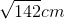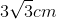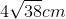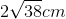Explanation:

The formula for the relationship between diagonals and sides of a parallologram is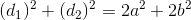,

where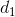represents one diagonal,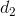represents the other diagonal,represents a side, andrepresents the adjoining side.

So, in this problem, substitute the known values and solve for the missing diagonal.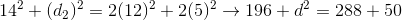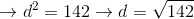So, the missing diagonal is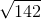cm.

### Example Question #2 : How To Find The Length Of The Diagonal Of A Parallelogram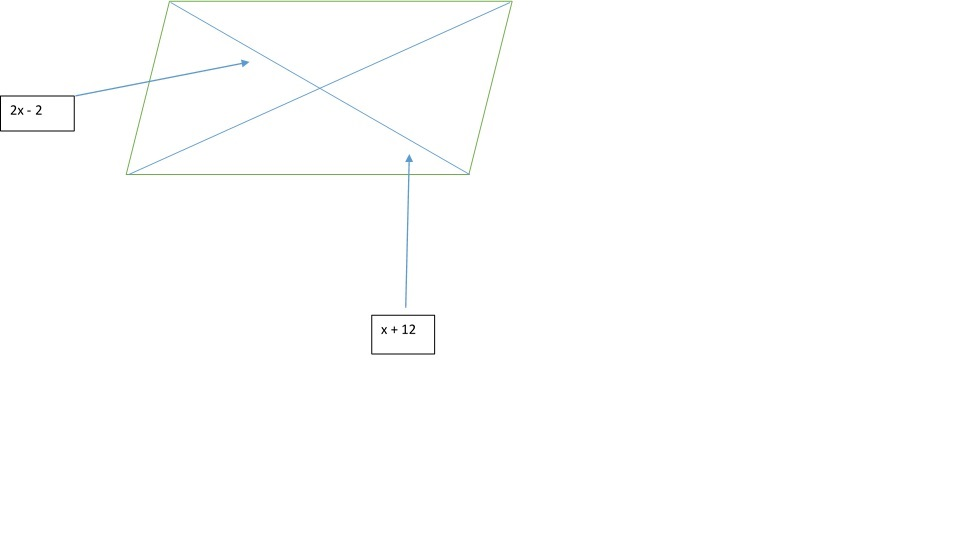In the parallogram above, find the length of the labeled diagonal.Explanation:

In a parallogram, diagonals bisect one another, thus you can set the two segments that are labeled in the picture equal to one another, then solve for.

So,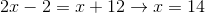.

If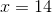, then you can substitute 14 into each labeled segment, to get a total of 52.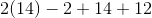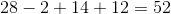### Example Question #3 : How To Find The Length Of The Diagonal Of A Parallelogram

In the parallogram below, find the length of the labeled diagonal.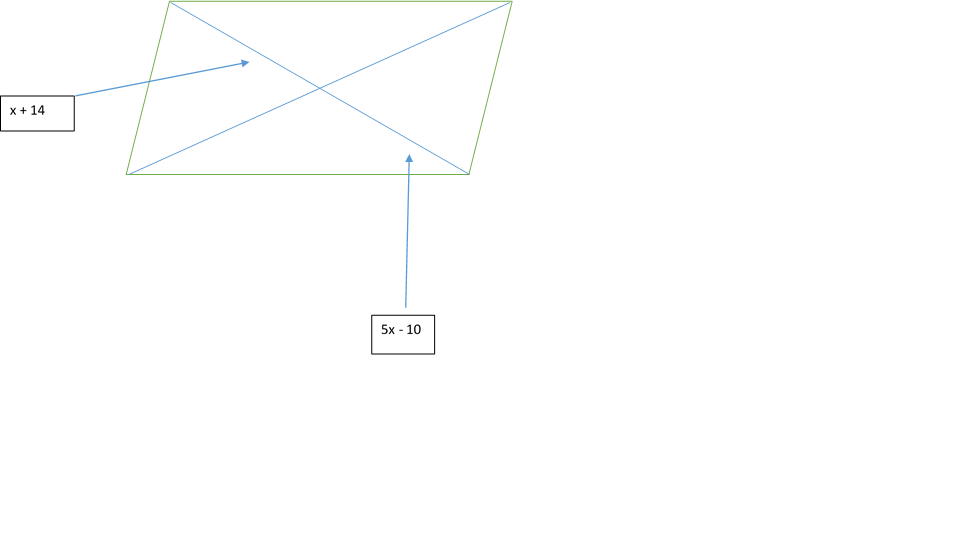Explanation:

In a parallelogram, the diagonals bisect one another, so you can set the labeled segments equal to each other and then solve for.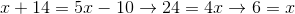.

If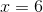, then you substitute 6 into each labeled segment, to get a total of 40.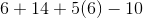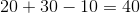### Example Question #4 : How To Find The Length Of The Diagonal Of A Parallelogram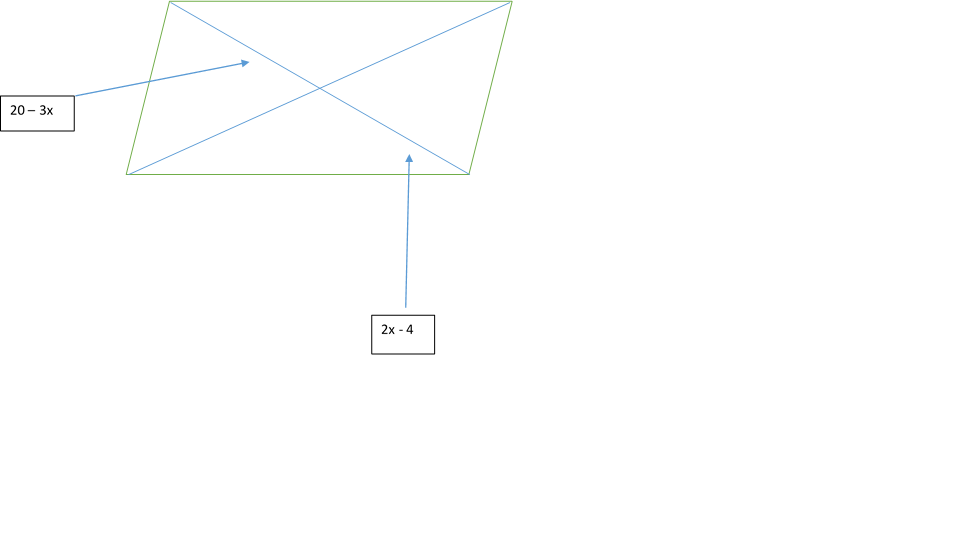In the parallelogram above, find the length of the labeled diagonal.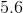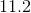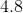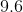Explanation:

In a parallelogram, the diagonals bisect each other, so you can set the labeled segments equal to one another and then solve for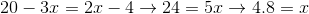.

Then, substitute 4.8 forin each labeled segment to get a total of 11.2 for the diagonal length.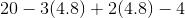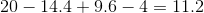### Example Question #5 : How To Find The Length Of The Diagonal Of A Parallelogram

Suppose a square has an area of 6.  What is the diagonal of the parallelogram?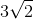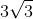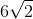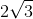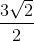Explanation:

Write the formula to find the side of the square given the area.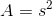Find the side.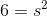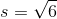The diagonal of the square can be solved by using the Pythagorean Theorem.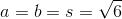Substitute and solve for the diagonal,.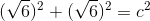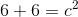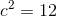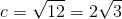### Example Question #6 : How To Find The Length Of The Diagonal Of A Parallelogram

If the side length of a square is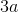, what is the diagonal of the square?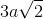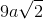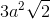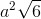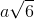Explanation:

Write the diagonal formula for a square.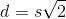Substitute the side length and reduce.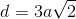### Example Question #7 : How To Find The Length Of The Diagonal Of A Parallelogram

Parallelogramhas diagonals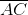and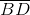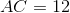and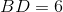.

True, false, or undetermined: Parallelogramis a rectangle.

False

Undetermined

True

False

Explanation:

One characteristic of a rectangle is that its diagonals are congruent. Since the diagonals of Parallelogramare of different lengths, it cannot be a rectangle.

### Example Question #8 : How To Find The Length Of The Diagonal Of A Parallelogram

Parallelogramhas diagonalsandand.

True, false, or undetermined: Parallelogramis a rhombus.

True

Undetermined

False